# Accuplacer Advanced Algebra & Functions Practice Test

The most difficult of the Next-Generation Accuplacer math test covers Advanced Algebra & Functions (AAF). Try our free Accuplacer Advanced Algebra practice test to review these topics. This test includes 20 multiple choice questions along with detailed answer explanations.

ACCUPLACER AAF Test Prep

If you are serious about getting a great score on your Accuplacer AAF test, try out our recommended Accuplacer AAF Prep Course.

Congratulations - you have completed .

You scored %%SCORE%% out of %%TOTAL%%.

Your performance has been rated as %%RATING%%

 Question 1

### The length of a rectangle is four more than its width. If the area of the rectangle is 12, find the length of the rectangle.

 A $-6$ B $2$ C $4.5$ D $6$
Question 1 Explanation:
The correct choice is (D). Since the length of the rectangle is four more than the rectangle’s width, we can write $l$ as $w + 4$.

Substituting in $w + 4$ for $l$ and $12$ for $A$ into the equation $A = l × w$, we get $12 = w(w + 4)$.

Simplifying, we get $12 = w^2 + 4w$.

Subtracting $12$ from both sides, we get $0 = w^2 + 4w - 12$, which can be factored as $(w + 6)(w - 2) = 0$.

Since $w$ must be positive, we equate $w - 2$ to $0$ to get $w = 2$. Since $l = w + 4$, we find that $l = 6$.
 Question 2

###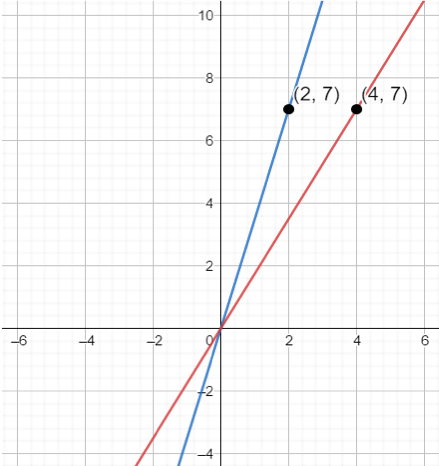The graph above shows a cost comparison for two different taxi services. The $x$-axis of the graph represents the number of kilometers driven, and the $y$-axis represents the taxi fare in dollars. The red line pictured above represents Taylor’s taxi service and the blue line represents Chandan’s carpool service. How much more does Chandan’s carpool service cost than Taylor’s taxi service per kilometer?

 A $\$1.75$B$\$2.00$ C $\$3.50$D$\$7.00$
Question 2 Explanation:
The correct choice is (A). We first find the equation of Chandan’s taxi service. First we find the slope, which is equal to:

$\dfrac{7 - 0 \, \text{ dollars}}{2 - 0 \, \text{ kilometers}}$ $= 3.5 \dfrac{\$}{\text{km}}\text{or } \$3.5 \text{ per km}$

Next, we find the equation of Taylor’s taxi service. We find the slope, which is equal to:

$\dfrac{7 - 0 \, \text{ dollars}}{4 - 0 \, \text{ kilometers}}$ $= 1.75 \dfrac{\$}{\text{km}}\text{or } \$1.75 \text{ per km}$

To find how much more Chandan’s taxi service costs than Taylor’s taxi service, we find $3.5 - 1.75 = \$1.75$per kilometer.  Question 3 ###$f(x) = 5x(x + 3)$. Which of the following is$f(x − 3)$?  A$5x^2 − 15x$B$0$C$5x^2$D$5x^2 + 15x$Question 3 Explanation: The correct choice is (A). To find$f(x − 3)$, we need to substitute$(x − 3)$for$x$.$f(x) = 5x(x + 3)f(x − 3) = 5(x − 3)((x − 3) + 3)f(x − 3) = 5(x − 3)(x − 3 + 3)f(x − 3) = 5(x − 3)(x)f(x − 3) = (5x − 15)(x)f(x − 3) = 5x^2 − 15x$ Question 4 ### Which of the following is equivalent to$(8^x)^{3x} = 24$where$x ≥ 0$?  A$x = \dfrac{\log_8 24}{4}$B$x = \dfrac{3}{4}$C$x = \sqrt{\dfrac{\log_8 24}{3}}$D$x = \sqrt{\dfrac{\log_3 24}{8}}$Question 4 Explanation: The correct choice is (C). The equation$(8^x)^{3x} = 24$can be simplified to$8^{3x^2} = 24$. We can rewrite this as:$\require{cancel} \cancel{\log_8}(\cancel{8}^{3x^2}) = \log_8⁡ (24)3x^2 = \log_8⁡ 24$Solving for$x$, we get:$x = \sqrt{\dfrac{\log_8 24}{3}}$ Question 5 ###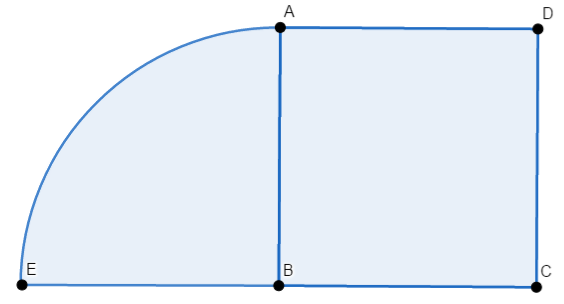Square$ABCD$has an area of$16 \text{m}^2$. In sector$AE$,$AB ≅ EB$. What is the total perimeter of the figure above?  A$2π + 20$B$2π + 16$C$8π + 16$D$8π + 20$Question 5 Explanation: The correct choice is (B). Since square$ABCD$has an area of$16 \text{m}^2$, it has a side length of$4\text{m}$. Therefore:$AD + DC + CB + BE= 4 × 4 = 16$Next, we find the perimeter of the quarter-circle which is equivalent to:$\dfrac{2π(4)}{4} = 2π$Summing up, the perimeter of the entire figure is$2π + 16$.  Question 6 ### Which of the following is the correct factorization of$5x^2 − 15x − 50$?  A$(5x + 5)(x − 10)$B$5(x − 5)(x + 2)$C$(5x − 5)(x + 10)$D$5(x − 2)(x + 5)$Question 6 Explanation: The correct choice is (B). When factoring quadratics, begin by factoring out any common factor of the coefficients:$5(x^2 − 3x − 10)$Then factor the "simplified" quadratic:$5(x − 5)(x + 2)$ Question 7 ### Which of the following is equivalent to$f(x) = \dfrac{x^2 + 2x − 15}{x^2 − x − 6}$as long as$x ≠ 3$and$x ≠ − 2$?  A$\dfrac{x − 3}{x + 2}$B$\dfrac{x + 5}{x − 3}$C$\dfrac{x − 3}{x − 6}$D$\dfrac{x + 5}{x + 2}$Question 7 Explanation: The correct choice is (D). As a general rule, when dealing with rational functions, begin by factoring the numerator and denominator.$\dfrac{(x + 5)(x − 3)}{(x − 3)(x + 2)}= \require{cancel} \dfrac{(x + 5) \cancel{(x − 3)}}{(\cancel{x − 3})(x + 2)}= \dfrac{x + 5}{x + 2}$ Question 8 ### What are the solutions to the following equation?$(2x + \sqrt{2})(5x + \sqrt{19})= 0$ A$x = \dfrac{-\sqrt{2}}{2}\text{ and }\dfrac{-\sqrt{19}}{5}$B$x = \dfrac{\sqrt{2}}{2}\text{ and }\dfrac{\sqrt{19}}{5}$C$x = \dfrac{-\sqrt{2}}{2}\text{ and }\dfrac{\sqrt{19}}{5}$D$x = \dfrac{\sqrt{2}}{2}\text{ and }\dfrac{-\sqrt{19}}{5}$Question 8 Explanation: The correct choice is (A). Using the zero product property, we set$(2x + \sqrt{2})$equal to$0$and$(5x + \sqrt{19})$equal to$0$. Solving$2x + \sqrt{2} = 0$, we get:$x = \dfrac{-\sqrt{2}}{2}$Solving$5x + \sqrt{19} = 0$, we get:$x = \dfrac{-\sqrt{19}}{5}$Therefore, the zeroes of the equation are:$x = \dfrac{-\sqrt{2}}{2}\text{ and }\dfrac{-\sqrt{19}}{5}$ Question 9 ###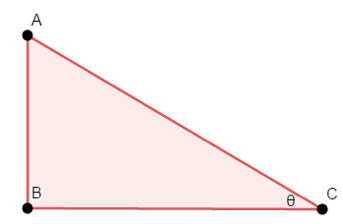Right triangle$ABC$is shown above. Benedict uses the equation$\tan^{-1}⁡(\frac{8}{15})$to find the angle measure of$θ$. What is the value of$\sin⁡ θ + \cos ⁡θ$?  A$\dfrac{23}{17}$B$\dfrac{7}{15}$C$\dfrac{8}{15}$D$\dfrac{15}{17}$Question 9 Explanation: The correct choice is (A). Since the tangent of a triangle can be found by dividing the length of the side opposite to the angle$θ$by the length of the side adjacent to the angle$θ$. The problem states that$\tan⁡ θ = \frac{8}{15}$, which means that$AB = 8$and$BC = 15$. The problem also states that the problem is a right triangle, which means that$AC = \sqrt{8^2 + 15^2} = 17$via the Pythagorean theorem. Next, we find:$\sin⁡ θ =\dfrac{ \text{Length of side opposite to } θ}{\text{Length of hypotenuse}}= \dfrac{8}{17}\cos⁡ θ =\dfrac{\text{Length of side adjacent to } θ}{\text{Length of hypotenuse}}= \dfrac{15}{17}$Adding$\sin ⁡θ$to$\cos ⁡θ$, we get:$\dfrac{8}{17} + \dfrac{15}{17} = \dfrac{23}{17}$ Question 10 ###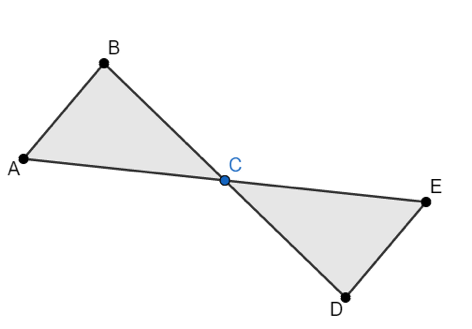Suppose$AC ≅ EC$. Which of the following must be true in order to prove that triangles$ABC$and$EDC$are congruent?  A$AB ≅ ED$B$∠ACB ≅ ∠ECD$C$CD ≅ CE$D$BC ≅ DC$Question 10 Explanation: The correct choice is (D). First, we know that$∠BCA ≅ ∠DCE$since they are vertical angles. We are also given that$AC ≅ EC$. Looking at choice (D), we see that if$BC ≅ DC$, triangles$ABC$and$EDC$would be congruent using the Side-Angle-Side postulate.  Question 11 ### If$x > 0$and$y > 0$, which of the following expressions is equivalent to$\dfrac{\sqrt{x} + \sqrt{y}}{xy}$?  A$\dfrac{\sqrt{x + y}}{xy}$B$\dfrac{x + y}{\sqrt{x + y}}$C$\dfrac{1}{y\sqrt{x}} + \dfrac{1}{x\sqrt{y}}$D$\dfrac{1}{\sqrt{xy}}$Question 11 Explanation: The correct choice is (C). The expression$\dfrac{\sqrt{x} + \sqrt{y}}{xy}$can be separated into:$\dfrac{\sqrt{x}}{xy} + \dfrac{\sqrt{y}}{xy}$This expression can be rewritten as:$\dfrac{x^{\frac{1}{2}}}{xy} + \dfrac{y^{\frac{1}{2}}}{xy}= \dfrac{1}{(x^{1 − \frac{1}{2}})(y)}+ \dfrac{1}{(x)(y^{1 − \frac{1}{2}})}= \dfrac{1}{(x^{\frac{1}{2}})(y)}+ \dfrac{1}{(x)(y^{\frac{1}{2}})}= \dfrac{1}{y\sqrt{x}} + \dfrac{1}{x\sqrt{y}}$An alternate approach:$\dfrac{\sqrt{x}}{xy} + \dfrac{\sqrt{y}}{xy}= \dfrac{\cancel{\sqrt{x}}}{\cancel{\sqrt{x}} \sqrt{x} y} + \dfrac{\cancel{\sqrt{y}}}{x \cancel{\sqrt{y}}\sqrt{y}}= \dfrac{1}{\sqrt{x} y} + \dfrac{1}{x\sqrt{y}}$ Question 12 ### Which of the following equations has a slope parallel to$y = \dfrac{1}{2}(x + 3)$and passes through the point$(3, 16)$?  A$y = -2x + \dfrac{29}{2}$B$y = 2x + \dfrac{3}{2}$C$y = \dfrac{1}{2}x + \dfrac{29}{2}$D$y = -\dfrac{1}{2}x + \dfrac{3}{2}$Question 12 Explanation: The correct choice is (C). A line parallel to one with the equation of$y = \frac{1}{2}(x + 3)$has a slope of$\frac{1}{2}$. Applying the point-slope formula to find the equation of the line that passes through$(3, 16)$, we get$y - 16 = \frac{1}{2}(x - 3)$, which can be simplified to$y = \frac{1}{2}x + \frac{29}{2}$.  Question 13 ### Which of the following are the solutions to$3x^2 + 9x + 12 = 0$? Express$\sqrt{-1}$as i.  A$- \dfrac{3}{2} ± \dfrac{i\sqrt{7}}{2}$B$\dfrac{3}{2} ± \dfrac{3i\sqrt{7}}{2}$C$- \dfrac{3}{2} ± \dfrac{\sqrt{51}}{6}$D$\dfrac{3}{2} ± \dfrac{-i\sqrt{23}}{2}$Question 13 Explanation: The correct choice is (A). We can apply the quadratic formula to$3x^2 + 9x + 12 = 0$. Plugging in 3, 9, and 12 for$a$,$b$, and$c$respectively into:$\dfrac{-b ± \sqrt{b^2 - 4ac}}{2a}$, we get:$\dfrac{-9 ± \sqrt{-63}}{6}= \dfrac{-9 ± \sqrt{-1 × 9 × 7}}{6}= \dfrac{-9 ± \sqrt{9 × -1 × 7}}{6}= \dfrac{-9 ± \sqrt{9} \sqrt{-1} \sqrt{7}}{6}= \dfrac{-9 ± 3i \sqrt{7}}{6}= -\dfrac{9}{6} ± \dfrac{3i\sqrt{7}}{6}= -\dfrac{3}{2} ± \dfrac{i\sqrt{7}}{2}$ Question 14 ###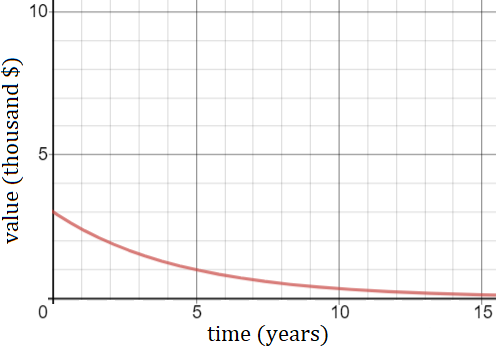The function above shows the value of a used bike over time. Which of the following is true about the function?  A$\text{The}\text{initial}\text{value}\text{of}\text{the}\text{bike}\text{is}\$300$ B $\text{The}$ $\text{value}$ $\text{of}$ $\text{the}$ $\text{bike}$ $\text{approaches}$ $\$0\text{as}\text{time}\text{progresses.}$C$\text{The}\text{value}\text{of}\text{the}\text{bike}\text{is}\text{stagnant}\text{after}\text{year}5.$D$\text{The}\text{total}\text{value}\text{of}\text{the}\text{bike}\text{is}15\text{thousand}\text{dollars.}$Question 14 Explanation: The correct choice is (B). Based on the graph, we see that initially (t = 0) the bike has a value of \$3,000. After 5 years (t = 5), the bike's value is approximately \$1,000. As time progresses, the bike’s value approaches \$0.
 Question 15

### Which of the following is true of the function shown below? $f(x) =$ $-0.7x^3 + 3.14x^2$ $+\, 6.28x + 121$

 A $\text{It}$ $\text{has}$ $\text{a}$ $\text{degree}$ $\text{of}$ $6,$ $\text{and}$ $\text{as}$ $x$ $\text{approaches}$ $∞,$ $y$ $\text{approaches}$ $-∞.$ B $\text{It}$ $\text{has}$ $\text{an}$ $\text{even}$ $\text{degree},$ $\text{and}$ $\text{as}$ $y$ $\text{approaches}$ $∞,$ $x$ $\text{approaches}$ $-∞.$ C $\text{It}$ $\text{has}$ $\text{a}$ $\text{degree}$ $\text{of}$ $3,$ $\text{and}$ $\text{as}$ $x$ $\text{approaches}$ $∞,$ $y$ $\text{approaches}$ $-∞.$ D $\text{It}$ $\text{has}$ $\text{a}$ $\text{prime}$ $\text{degree},$ $\text{and}$ $\text{as}$ $x$ $\text{approaches}$ $∞,$ $y$ $\text{approaches}$ $∞.$
Question 15 Explanation:
The correct choice is (C). Examining the function above, we know that its degree is odd and it has a negative leading coefficient.

In functions that have odd degrees and negative leading coefficients, as $x$ approaches ∞, $y$ approaches -∞, and as $x$ approaches -∞, $y$ approaches ∞. Thus, the only choice that correctly identifies the degree and the end behavior of the graph is choice C.
 Question 16

### Which of the following depicts a one-to-one function?

 A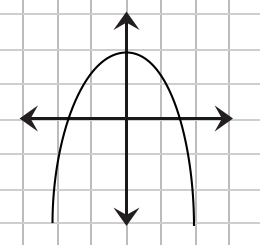B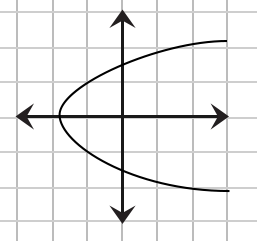C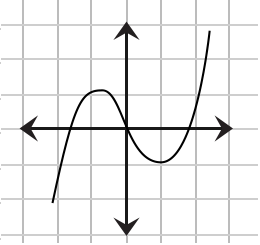D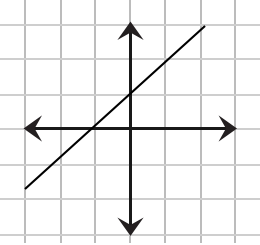Question 16 Explanation:
The correct choice is (D). To be a function, the graph of the relation must pass the vertical line test. Graph (B) fails the vertical line test, but (A), (C), and (D) pass.

To be a one-to-one function, the graph of the function must pass the horizontal line test. Only graph (D) passes.
 Question 17

###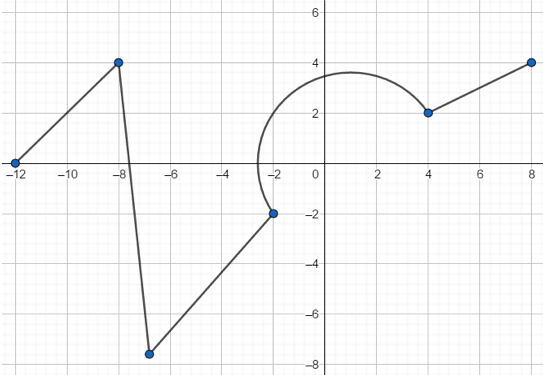Relation $z$ is pictured above. What is the value of $z(z(-8))$?

 A $8$ B $4$ C $2$ D $-2$
Question 17 Explanation:
The correct choice is (C). First, we find the value of $z(-8)$ which is $4$. Next, we plug $4$ back into the function. We are left to find $z(4)$ which is equal to $2$.
 Question 18

### For an acute angle, $x$, which of the following is guaranteed to be greater than $1$?

 A $\sin(x)$ B $\cos(x)$ C $\tan(x)$ D $\dfrac{1}{\cos(x)}$
Question 18 Explanation:
The correct choice is (D). $\sin(x)$ and $\cos(x)$ can never be greater than $1$, regardless of the angle.

$\tan(x)$ will exceed $1$ for acute angles exceeding $45°$, but will be less than $1$ for acute angles less than $45°$.

$\cos(x)$ will be less than $1$ for all acute angles. This means that $\dfrac{1}{\cos(x)}$ is guaranteed to be greater than $1$ for the same angles.

For example, the $\cos(30°) = \dfrac{1}{2}$, therefore $\dfrac{1}{\cos(30°)} = \dfrac{1}{\frac{1}{2}}$ $= 1 \cdot \dfrac{2}{1} = 2$.
 Question 19

### Which of the following provides the domain of $y = \dfrac{37}{\sqrt{x + 2}}$?

 A $x > 0$ B $x > −2$ C $x < −2$ D $x < 2$
Question 19 Explanation:
The correct choice is (B). The domain represents all values of $x$, the input, for which a value of $y$, the output, exists.

We cannot have a negative under a square root and we cannot divide by $0$.

This means $x + 2 > 0$ and, when cancelling out the $2$ by subtracting it from both sides, $x > −2$.
 Question 20

### A quadratic function has roots at $(-3, 0)$ and $(5, 0)$. Which of the following could represent the minimum point of the function?

 A $(1, -16)$ B $(1.5, -16)$ C $(2, -16)$ D $(1, 0)$
Question 20 Explanation:
The correct choice is (A). We are given that the two zeroes of the graph are $(-3, 0)$ and $(5, 0)$, which means that the minimum point of the function has an $x$ value of $\dfrac{-3 + 5}{2} = 1$.

The equation of this parabola is $y = (x + 3)(x - 5)$ from the given roots. This means the $y$-value of the parabola at $x = 1$ is $(1 + 3)(1 - 5) = -16$. Therefore, the correct answer is $(1, -16)$.
Once you are finished, click the button below. Any items you have not completed will be marked incorrect.
There are 20 questions to complete.
 ← List →